# Rolling And Extrusion ME 482 Manufacturing Systems Rolling

• Slides: 17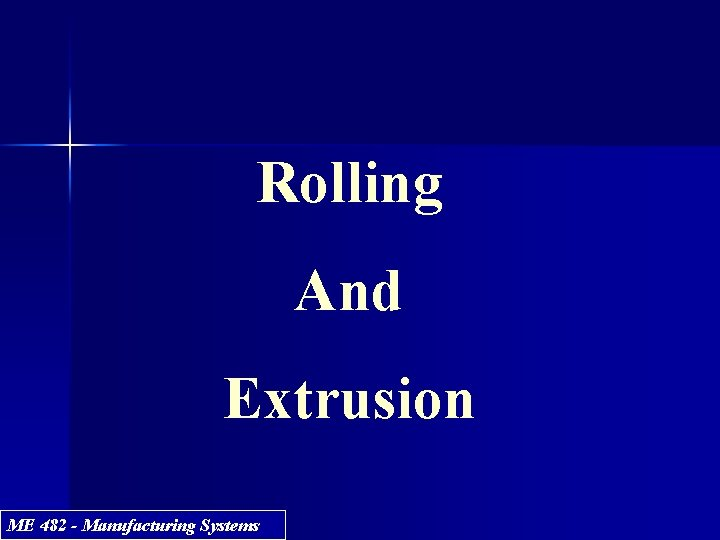Rolling And Extrusion ME 482 - Manufacturing Systems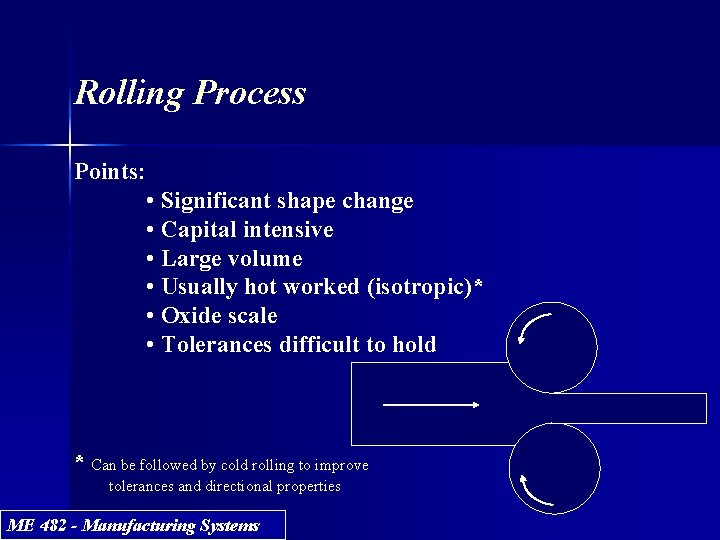Rolling Process Points: • Significant shape change • Capital intensive • Large volume • Usually hot worked (isotropic)* • Oxide scale • Tolerances difficult to hold * Can be followed by cold rolling to improve tolerances and directional properties ME 482 - Manufacturing SystemsRolling Products Billet Blooms (> 6” x 6”) Slab Ingots Billets (> 1. 5” x 1. 5”) Slab (> 10” x 1. 5”) ME 482 - Manufacturing Systems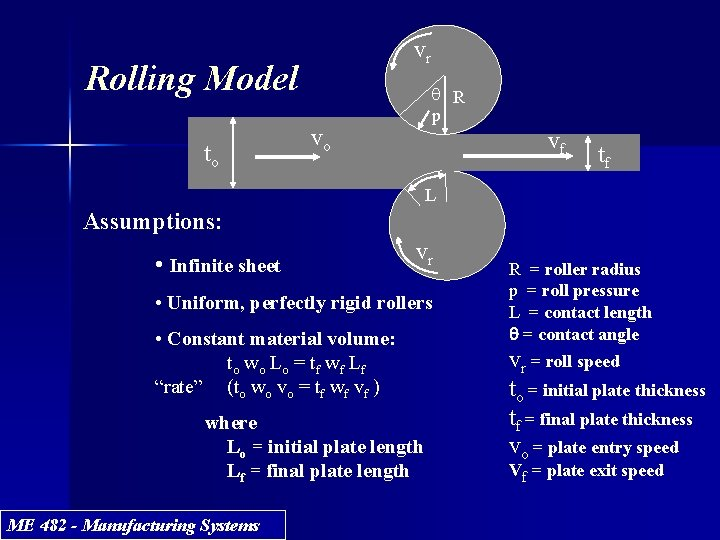vr Rolling Model to q R p vo vf tf L Assumptions: • Infinite sheet vr • Uniform, perfectly rigid rollers • Constant material volume: t o wo L o = t f wf L f “rate” (to wo vo = tf wf vf ) where Lo = initial plate length Lf = final plate length ME 482 - Manufacturing Systems R = roller radius p = roll pressure L = contact length q = contact angle vr = roll speed to = initial plate thickness tf = final plate thickness vo = plate entry speed Vf = plate exit speed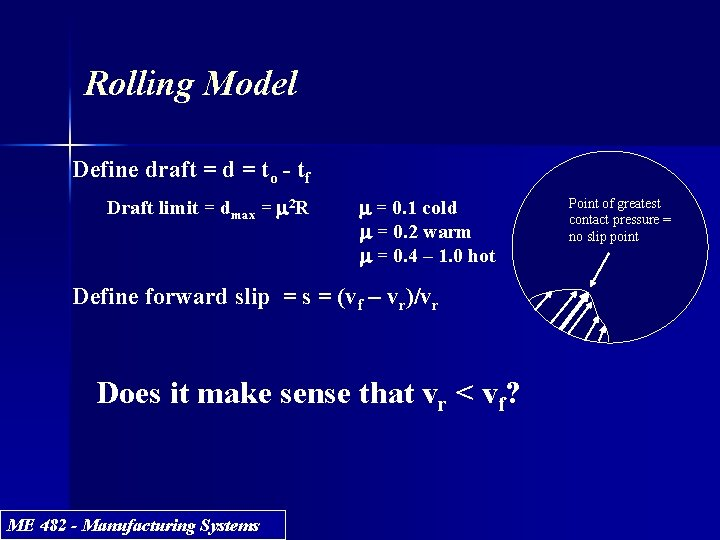Rolling Model Define draft = d = to - tf Draft limit = dmax = m 2 R m = 0. 1 cold m = 0. 2 warm m = 0. 4 – 1. 0 hot Define forward slip = s = (vf – vr)/vr Does it make sense that vr < vf? ME 482 - Manufacturing Systems Point of greatest contact pressure = no slip point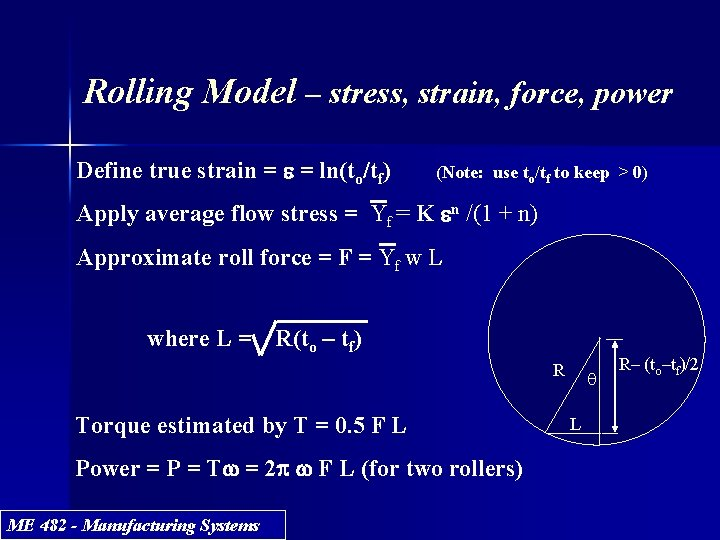Rolling Model – stress, strain, force, power Define true strain = e = ln(to/tf) (Note: use to/tf to keep > 0) Apply average flow stress = Yf = K en /(1 + n) Approximate roll force = F = Yf w L where L = R(to – tf) R Torque estimated by T = 0. 5 F L Power = P = Tw = 2 p w F L (for two rollers) ME 482 - Manufacturing Systems q L R– (to–tf)/2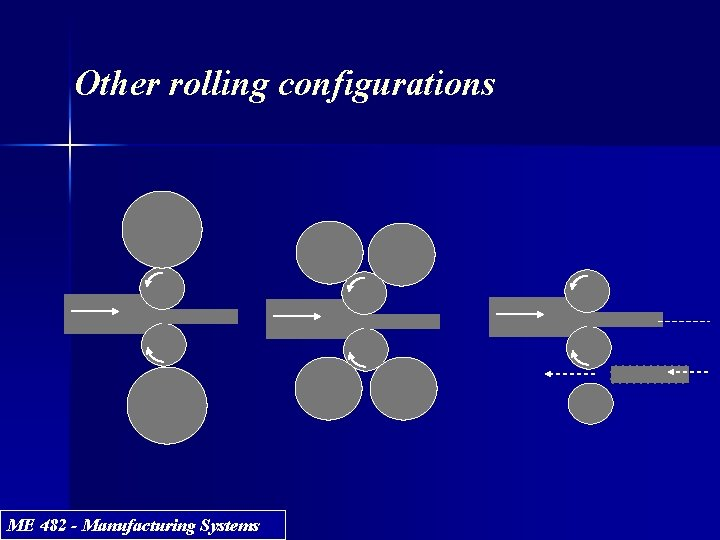Other rolling configurations ME 482 - Manufacturing SystemsExample 21. 1 in text Roll a 12 inch wide strip, that is 1 inch thick, to 0. 875 inch thickness in one pass with roll speed of 50 rpm and radius = 10 inches. Material has K = 40, 000 psi, n = 0. 15 and m = 0. 12. Determine if feasible and calculate F, T, and power if so. Solution: Feasible since dmax = (0. 12)2 (10) = 0. 144 in. > d = 1. 0 – 0. 875 = 0. 125 in. Contact length = L = 1. 118 in. e = ln(1. 0/0. 875) = 0. 134 Yf = (40, 000)(0. 134)0. 15/1. 15 = 25, 729 psi Rolling force = (25, 729)(12)(1. 118) = 345, 184 lb Torque = (0. 5)(345, 184)(1. 118) = 192, 958 in. -lb Power = P = (2 p)(50)(345, 184)(1. 118) = 121, 238, 997 in. -lb/min (306 hp) ME 482 - Manufacturing Systems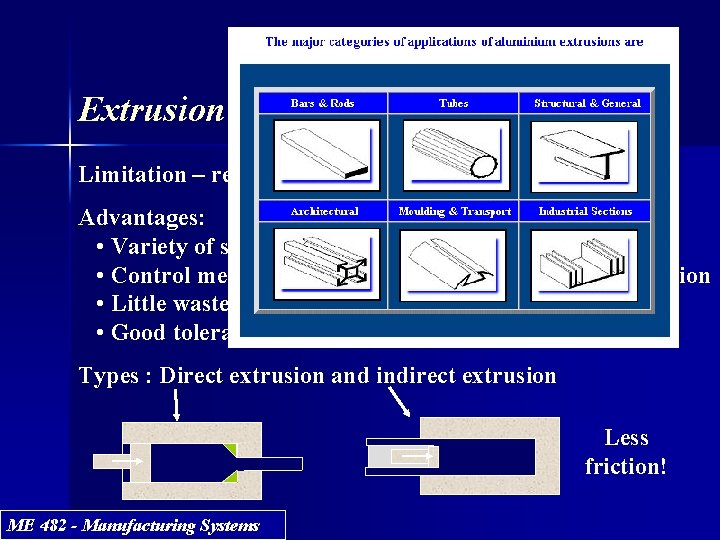Extrusion Limitation – requires uniform cross-section vs length Advantages: • Variety of shapes • Control mechanical properties in cold and warm extrusion • Little wasted material • Good tolerances Types : Direct extrusion and indirect extrusion Less friction! ME 482 - Manufacturing SystemsExtrusion Model Assumptions: L • Circular cross-section • Uniform stress distribution p Do p = ram pressure L = remaining billet length Do = chamber diameter Df = extrudate diameter ME 482 - Manufacturing Systems Df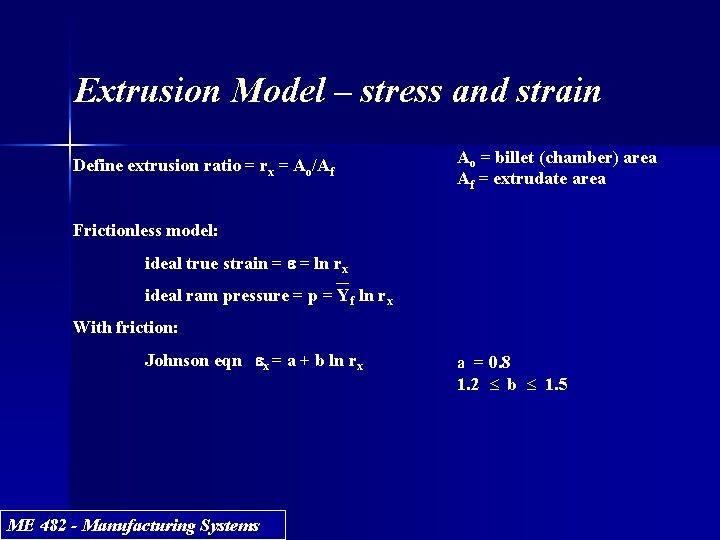Extrusion Model – stress and strain Define extrusion ratio = rx = Ao/Af Ao = billet (chamber) area Af = extrudate area Frictionless model: ideal true strain = e = ln rx ideal ram pressure = p = Yf ln rx With friction: Johnson eqn ex = a + b ln rx ME 482 - Manufacturing Systems a = 0. 8 1. 2 £ b £ 1. 5Extrusion Model – stress and strain Indirect extrusion ram pressure = p = Yf ex (ex is from Johnson eqn) and where Yf is found using the ideal true strain e = ln rx In direct extrusion, difficult to predict the chamber/billet interactive friction, so use the shear yield strength ( about Yf /2 ) to estimate the chamber wall shear force as pf p Do 2/4 = Yf p Do L/2 giving pf = 2 Y f L / Do and where pf = additional pressure to overcome wall friction force Total ram pressure becomes p = Yf (ex + 2 L / Do ) ME 482 - Manufacturing Systems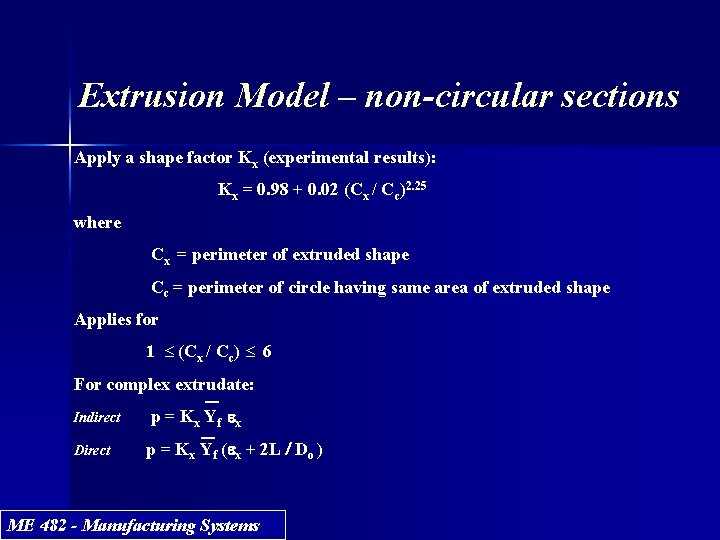Extrusion Model – non-circular sections Apply a shape factor Kx (experimental results): Kx = 0. 98 + 0. 02 (Cx / Cc)2. 25 where Cx = perimeter of extruded shape Cc = perimeter of circle having same area of extruded shape Applies for 1 £ (Cx / Cc) £ 6 For complex extrudate: Indirect Direct p = K x Yf e x p = Kx Yf (ex + 2 L / Do ) ME 482 - Manufacturing SystemsExtrusion Model – forces and power Ram force = F = p. Ao Power = P = Fv ME 482 - Manufacturing Systems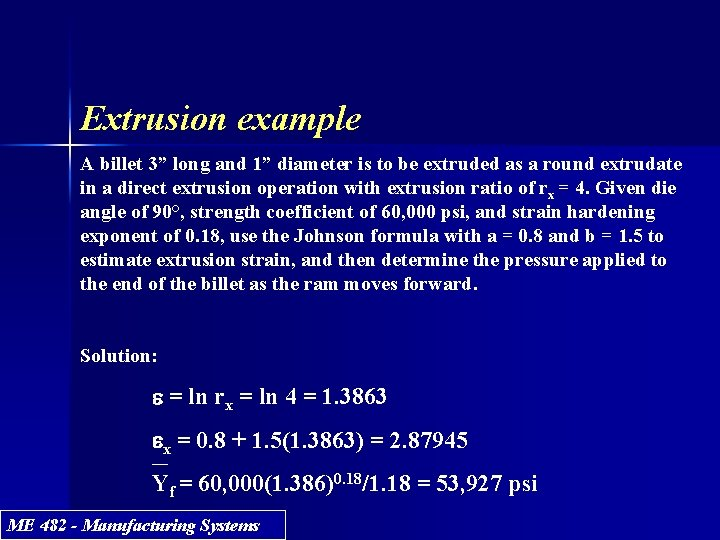Extrusion example A billet 3” long and 1” diameter is to be extruded as a round extrudate in a direct extrusion operation with extrusion ratio of rx = 4. Given die angle of 90°, strength coefficient of 60, 000 psi, and strain hardening exponent of 0. 18, use the Johnson formula with a = 0. 8 and b = 1. 5 to estimate extrusion strain, and then determine the pressure applied to the end of the billet as the ram moves forward. Solution: e = ln rx = ln 4 = 1. 3863 ex = 0. 8 + 1. 5(1. 3863) = 2. 87945 Yf = 60, 000(1. 386)0. 18/1. 18 = 53, 927 psi ME 482 - Manufacturing Systems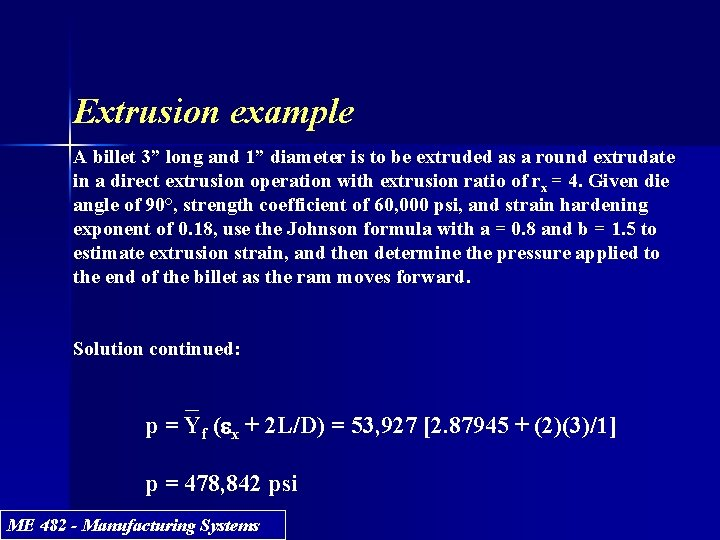Extrusion example A billet 3” long and 1” diameter is to be extruded as a round extrudate in a direct extrusion operation with extrusion ratio of rx = 4. Given die angle of 90°, strength coefficient of 60, 000 psi, and strain hardening exponent of 0. 18, use the Johnson formula with a = 0. 8 and b = 1. 5 to estimate extrusion strain, and then determine the pressure applied to the end of the billet as the ram moves forward. Solution continued: p = Yf (ex + 2 L/D) = 53, 927 [2. 87945 + (2)(3)/1] p = 478, 842 psi ME 482 - Manufacturing SystemsRolling and Extrusion What have we learned? ME 482 - Manufacturing Systems